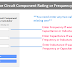Here, in this LC Resonance Circuit(or LC Tank Circuit) Calculator, you can calculate the component rating of an LC resonance circuit or you can calculate the resonance frequency when you have the component rating. This calculator allows you to perform calculations with different Units.

LC Resonance Circuit Component and Frequency Calculator

## LC Resonance Circuit Component and Frequency Calculator

Enter any two values to get the missing one

 Frequency: Hz kHz MHz Capacitance: F pF nF uF mF Inductance: H mH uH

### How to Use This Calculator?

To use the above "LC Resonance Circuit Component and Frequency Calculator," follow the below steps,

1. Enter any two known values (Frequency, Capacitance, or Inductance) into the corresponding input fields. You can leave the other input fields blank.

For example, you have the frequency and inductance then enter these values in the respective input fields. The calculator will give you the capacitance value.

2. Select the appropriate units for the entered values using the dropdown menus.

3. Click the "Calculate" button to find the missing value based on the entered information. The calculator will compute the missing value for you.

4. If you want to start over or make new calculations, click the "Reset" button to clear the input fields and start fresh.

### Formula

Here are the formulas, the calculator uses to perform calculations,

Resonant Frequency Calculation Formula:

F = 1 / (2 * π * √(L * C))

Inductance Calculation Formula:

L = 1 / (4 * π^2 * F^2 * C)

Capacitance Calculation Formula:

C = 1 / (4 * π^2 * F^2 * L)

Where:

F is the frequency of the LC resonance circuit.
C is the capacitance of the capacitor in the LC circuit.
L is the inductance of the inductor in the LC circuit.

### Example

Now, let's take examples to understand the actual calculation.

Resonant Frequency Calculation:

Suppose we have an LC resonance circuit with an inductor (L) having a value of 100 mH (millihenries) and a capacitor (C) with a value of 1 µF (microfarad). We want to find the resonant frequency (F) of this circuit.

First, convert the values to their base units:

L = 100 mH = 100 * 1e-3 H = 0.1 H (Henry)
C = 1 µF = 1 * 1e-6 F = 1e-6 F (Farad)

Now, calculate the resonant frequency (F),

F = 1 / (2 * π * √(0.1 * 1e-6))

F ≈ 503 Hz

So, the resonant frequency of this LC circuit with an inductance of 100 mH and a capacitance of 1 µF is approximately 503 Hz.

Inductance Calculation:

Suppose we have an LC resonance circuit with a resonant frequency (F) of 2 kHz and a capacitor (C) with a value of 10 nF (nanofarads). We want to find the inductance (L) of the inductor in this circuit.

First, convert the values to their base units,

F = 2 kHz = 2 * 1e3 Hz = 2000 Hz
C = 10 nF = 10 * 1e-9 F = 1e-8 F (Farad)

Now, calculate the inductance (L),

L = 1 / (4 * π^2 * (2000)^2 * 1e-8)

L ≈ 0.633 mH

So, the inductance of the inductor in this LC circuit with a resonant frequency of 2 kHz and a capacitance of 10 nF is approximately 0.633 millihenries (mH).

Capacitance Calculation:

Suppose we have an LC resonance circuit with a resonant frequency (F) of 1 MHz and an inductor (L) with a value of 500 µH (microhenries). We want to find the capacitance (C) of the capacitor in this circuit.

First, convert the values to their base units,

F = 1 MHz = 1 * 1e6 Hz = 1e6 Hz
L = 500 µH = 500 * 1e-6 H = 5e-4 H (Henry)

Now, calculate the capacitance (C),
C = 1 / (4 * π^2 * (1e6)^2 * 5e-4)

C ≈ 0.0507 nF

So, the capacitance of the capacitor in the LC circuit with a resonant frequency of 1 MHz and an inductance of 500 µH is approximately 0.0507 nanofarads (nF).

Thank you for visiting the Website. Keep visiting for more Updates.

LC Resonance Circuit Calculator (Component Rating, Frequency)Reviewed by Author on July 26, 2023 Rating: 5